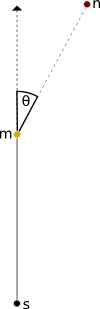# Implementing HTML5 Canvas Polyline Simplification

### Or, fun with vector geometry and state machines!

Once again, I’m toying around with HTML5 canvas. As part of the larger project, I needed a way to generate simplified paths based on mouse drags where ‘simplified paths’ means polyline paths with as few internal points as possible to accurately express the movement of the mouse. I did a bit of research and came up with a bunch of papers on the topic, both for purely polyline simplification as well as polycurve simplification, but I decided it would be more fun to roll my own.

So I cranked up some math rock, busted out the notebook, and dusted off the brain cells dedicated to vector geometry. The breakthrough is realizing that the algorithm can run at a single point on a time. Here is the resulting algorithm in pseudocode:

1. Initialize TMIN, LMIN, line_list = [].
2. Save the mousedown point (s). Push s onto line_list.
3. Save the point after the first mousemove (m). Push m onto line_list.
4. For each new point, n (i.e. on mousemove event):
1. Let p = n – m.
2. If ||p|| < LMIN:
1. Pop the last element from line_list.
2. Push n onto line_list.
3. Return.
3. Else:
1. Let o = m - s.
2. Let θ = arccos(o . p / (||o|| * ||p||)).
3. If θ < TMIN:
1. Pop the last element from line_list.
2. Push n onto line_list.4.
4. Else:
1. Push n onto line_list.
2. Let s = m.
5. Let m = n.

At the end, line_list is the simplified list. Here is a diagram of the relevant variables:In normal words, the algorithms only include points that are close enough to the line established by the previous two points, assuming that the mouse has gone far enough away from the last point. The nice thing about this method relative to some more complex ones (e.g., least-squares fit to the original line) is that the algorithm only needs to hold the points of the resulting line and the latest point.

So DOES it work? You can give it a go here. Fork the code (Coffeescript) here.